# Convex Shapes

Convex Shapes

 1 Convex Definition 2 Convex Polygon 3 Concave Definition 4 Differences between Convex and Concave Shapes (with illustration) 5 Thinking out of the Box! 6 Solved Examples on Convex Shapes 7 Important Notes on Convex Shapes 8 Practice Questions on Convex Shapes 9 Maths Olympiad Sample Papers 10 Frequently Asked Questions (FAQs)

We at Cuemath believe that Math is a life skill. Our Math Experts focus on the “Why” behind the “What.” Students can explore from a huge range of interactive worksheets, visuals, simulations, practice tests, and more to understand a concept in depth.

Book a FREE trial class today! and experience Cuemath's LIVE Online Class with your child.

## Convex Definition

A convex shape is a shape where all of its parts "point outwards."

In other words, no part of it points inwards.

For example, a full pizza is a convex shape as its full outline (circumference) points outwards.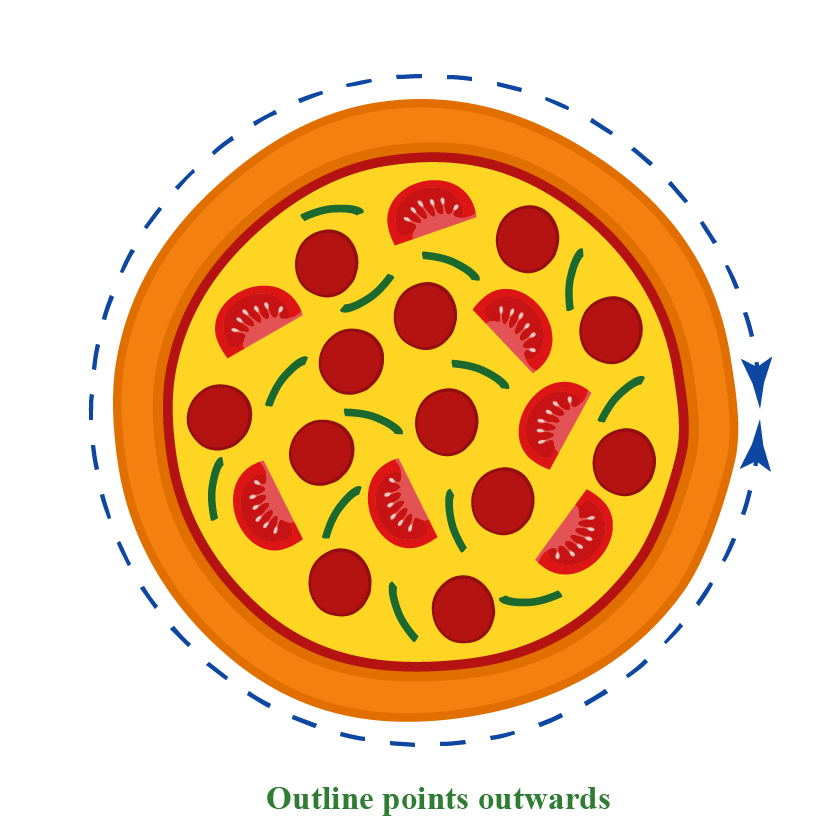Some other convex shapes are as follows:### Convex Definition in Geometry

A convex shape in Geometry is a shape where the line joining every two points of the shape lies completely inside the shape.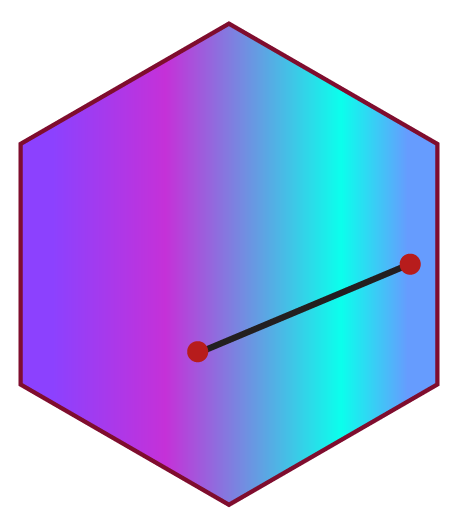### Convex Lens

A convex lens, as its name suggests, points outwards.

A convex lens is also known as "converging lens."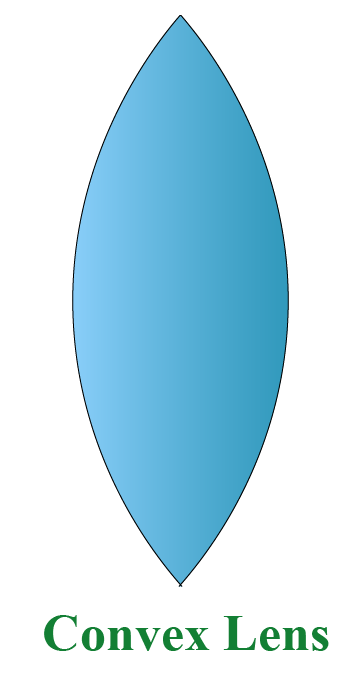## Convex Polygon

A convex polygon can be defined in three ways.

• A convex polygon is a polygon where all the vertices point inwards.
• A convex polygon is a polygon where all the interior angles are less than $$180^\circ$$.
• A convex polygon is a polygon where the line joining every two points of it lies completely inside it.

Some examples of convex polygons are as follows: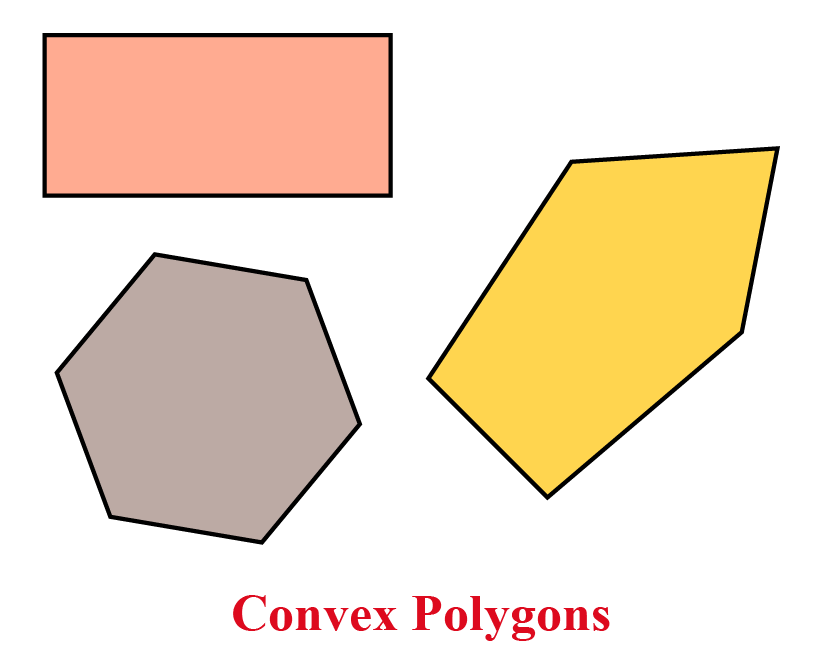In a convex polygon of $$n$$ sides, the formula for the sum of interior angles is as follows

 Sum of Interior Angles $$= 180 (n-2)^\circ$$

In a convex polygon of $$n$$ sides, the formula for the sum of exterior angles is as follows:

 Sum of Exterior Angles $$= 360^\circ$$

## Concave Definition

A concave shape is a shape where at least some portion of it "points inwards."

If we remove a slice of a pizza, it will look like this: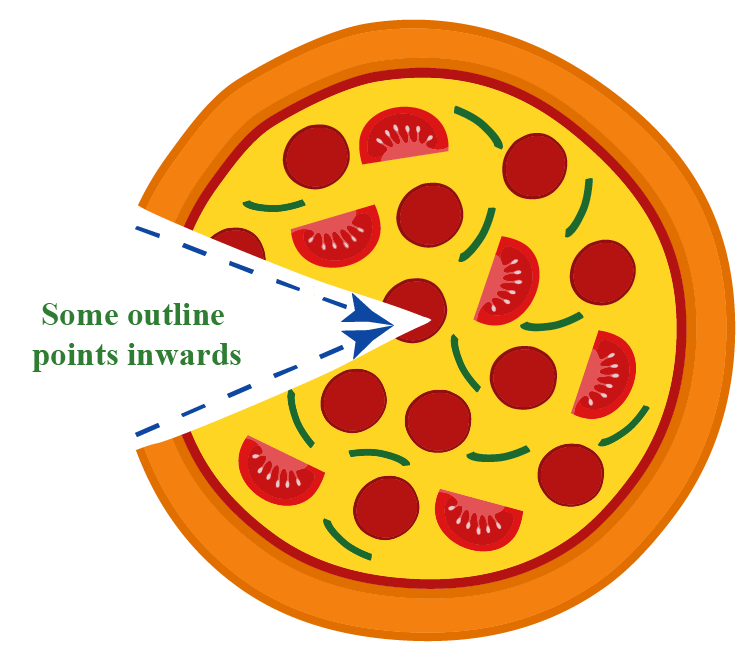In the image, some parts of the pizza's outline points inwards.

Hence, it is a concave shape.

Some other examples of concave shapes are as follows: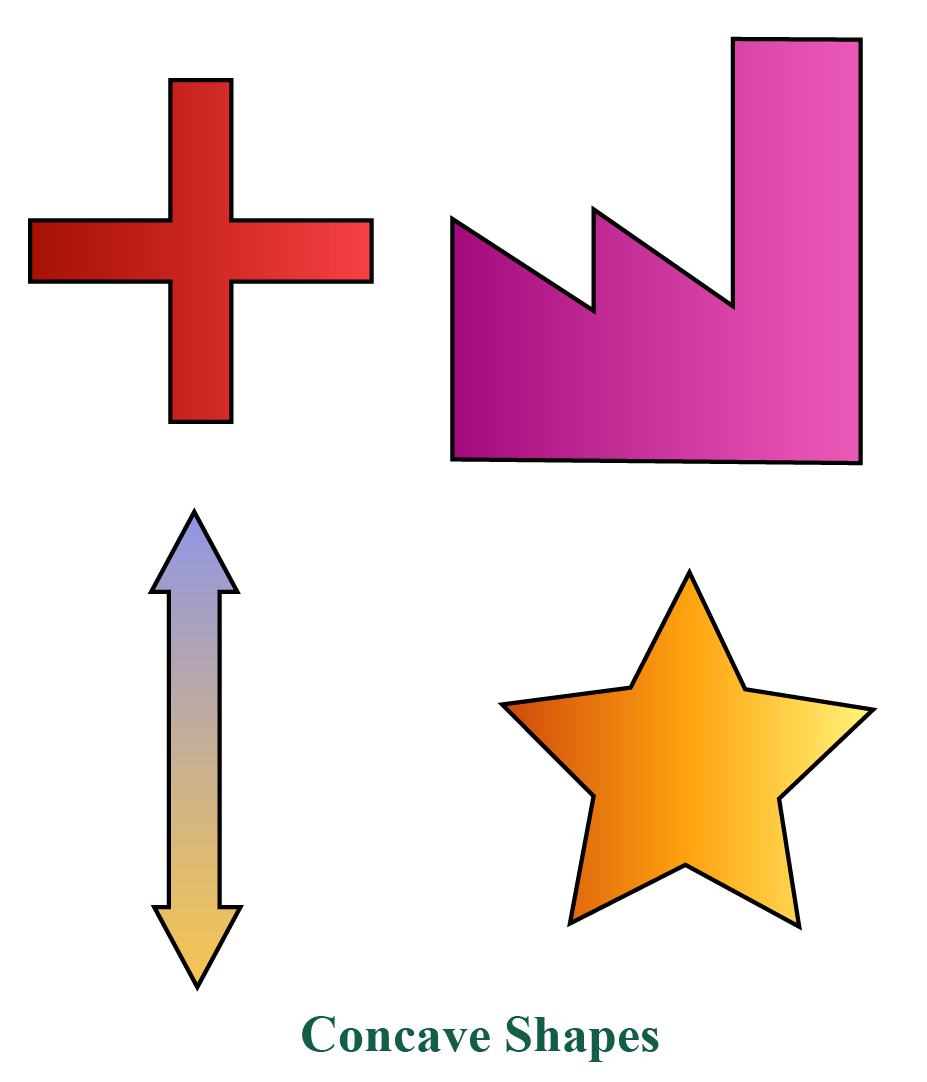CLUEless in Math? Check out how CUEMATH Teachers will explain Convex Shapes to your kid using interactive simulations & worksheets so they never have to memorise anything in Math again!

Explore Cuemath Live, Interactive & Personalised Online Classes to make your kid a Math Expert. Book a FREE trial class today!

## Differences Between Convex and Concave Shapes (With Illustration)

The differences between convex and concave shapes are as follows.

Convex Shapes Concave Shapes

The full outline of the convex shape points outwards. i.e., there are no dents.

Example: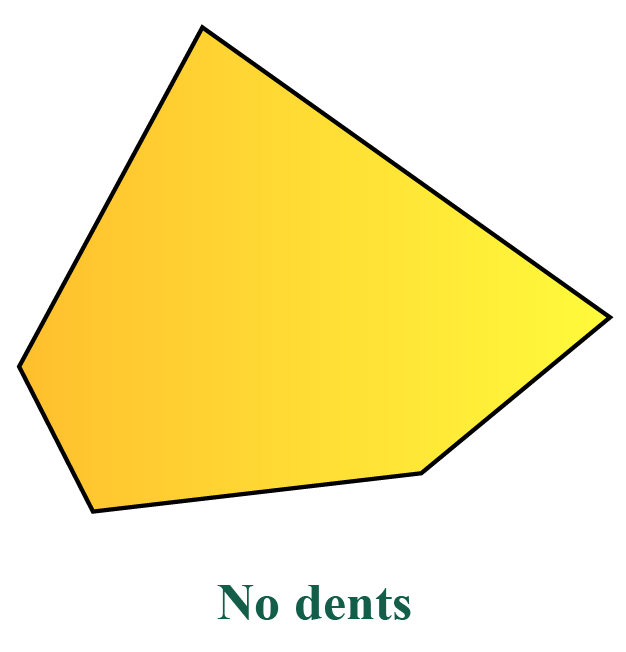At least some portion of the concave shape points outwards.i.e., there is a dent.

Example: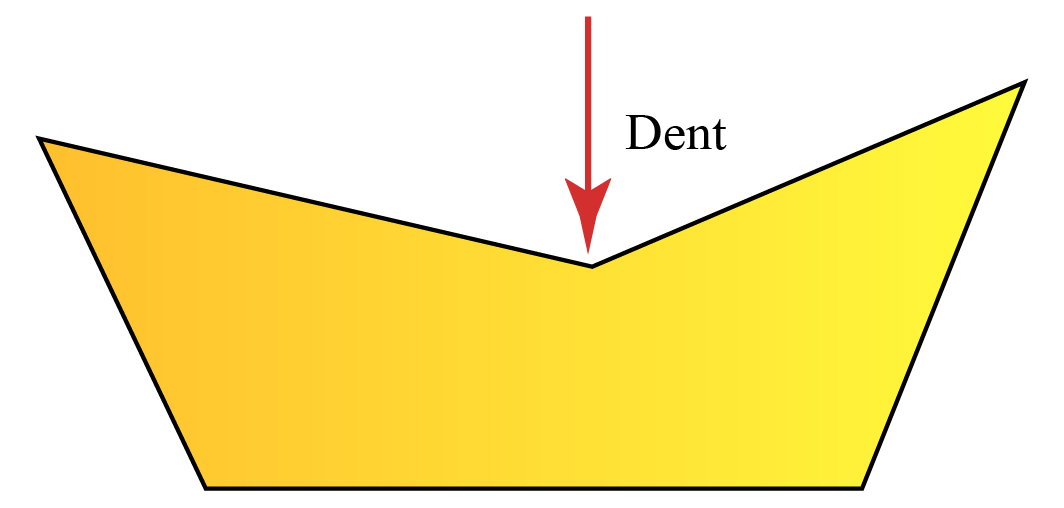All the interior angles of a convex polygon are less than $$180^\circ$$.

Example of a convex octagon: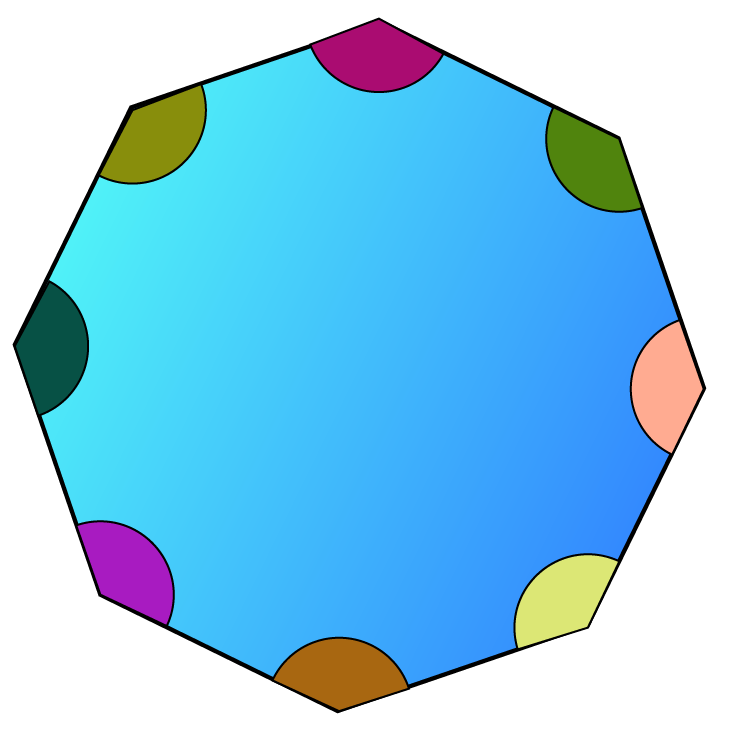At least one interior angle is greater than $$180^\circ$$.

Example of a concave octagon: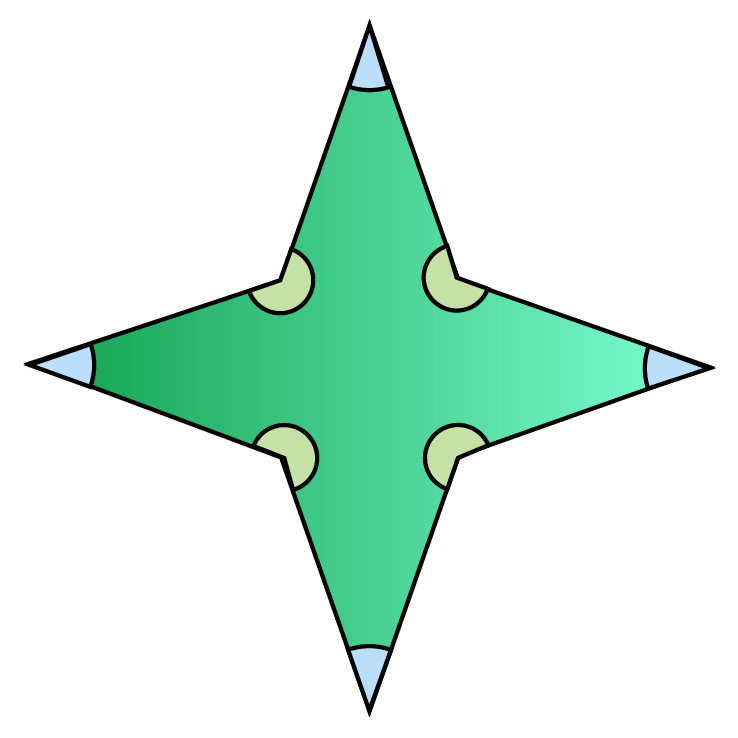The line joining any two points of the convex shape lies completely in it.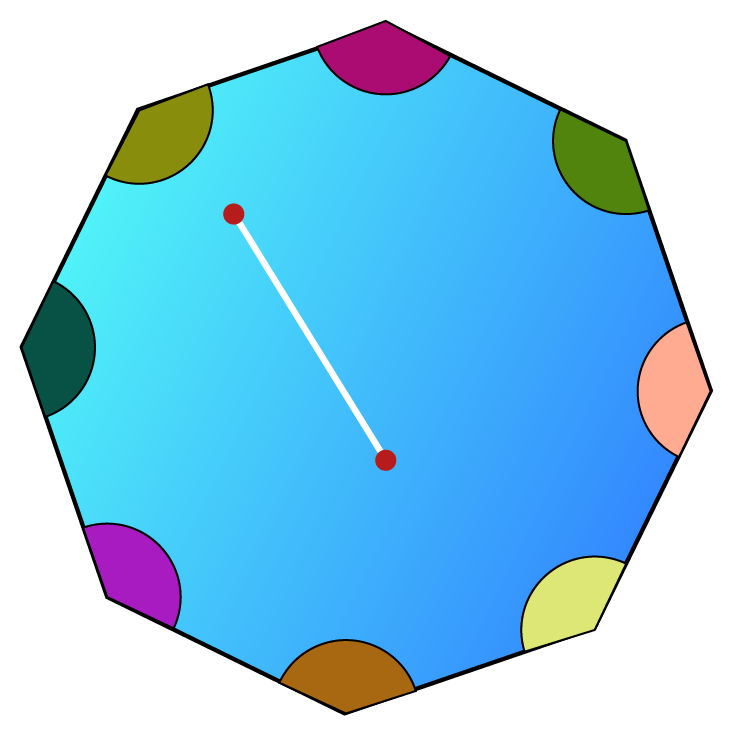The line joining any two points of the concave shape may or may not lie in it.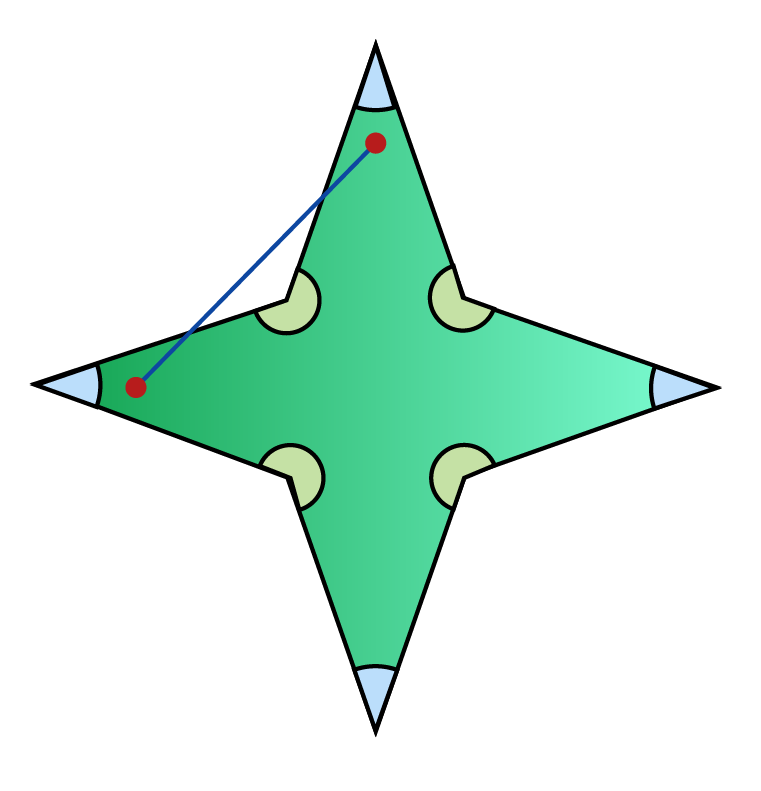You can identify the difference between the convex and the concave shapes by using the following illustration.

Here, you can drag the vertices of any polygon and see how it changes from "convex to concave" or vice versa.Think Tank
1. Is the sum of interior angles of a concave polygon of $$n$$ sides $$180(n-2)$$ degrees?
2. Is the sum of exterior angles of a concave polygon of $$n$$ sides $$360$$ degrees?

Help your child score higher with Cuemath’s proprietary FREE Diagnostic Test. Get access to detailed reports, customized learning plans, and a FREE counseling session. Attempt the test now.

## Solved Examples

 Example 1

What is the measure of an interior angle of a regular pentagon?

Solution:

The number of sides of a pentagon is, $$n=5$$

We know that the sum of all interior angles of a polygon of $$n$$ sides is $$180(n-2)$$ degrees.

Hence, the sum of the interior angles of the pentagon is:

$180^\circ(5-2) =180^\circ(3)=540^\circ$

Since the given pentagon is regular, all $$5$$ interior angles measure the same.

Therefore, the measure of each interior angle is:

$\dfrac{540^\circ}{5} = 108$

 Required angle $$=108^\circ$$
 Example 2

Identify the convex shapes among the following.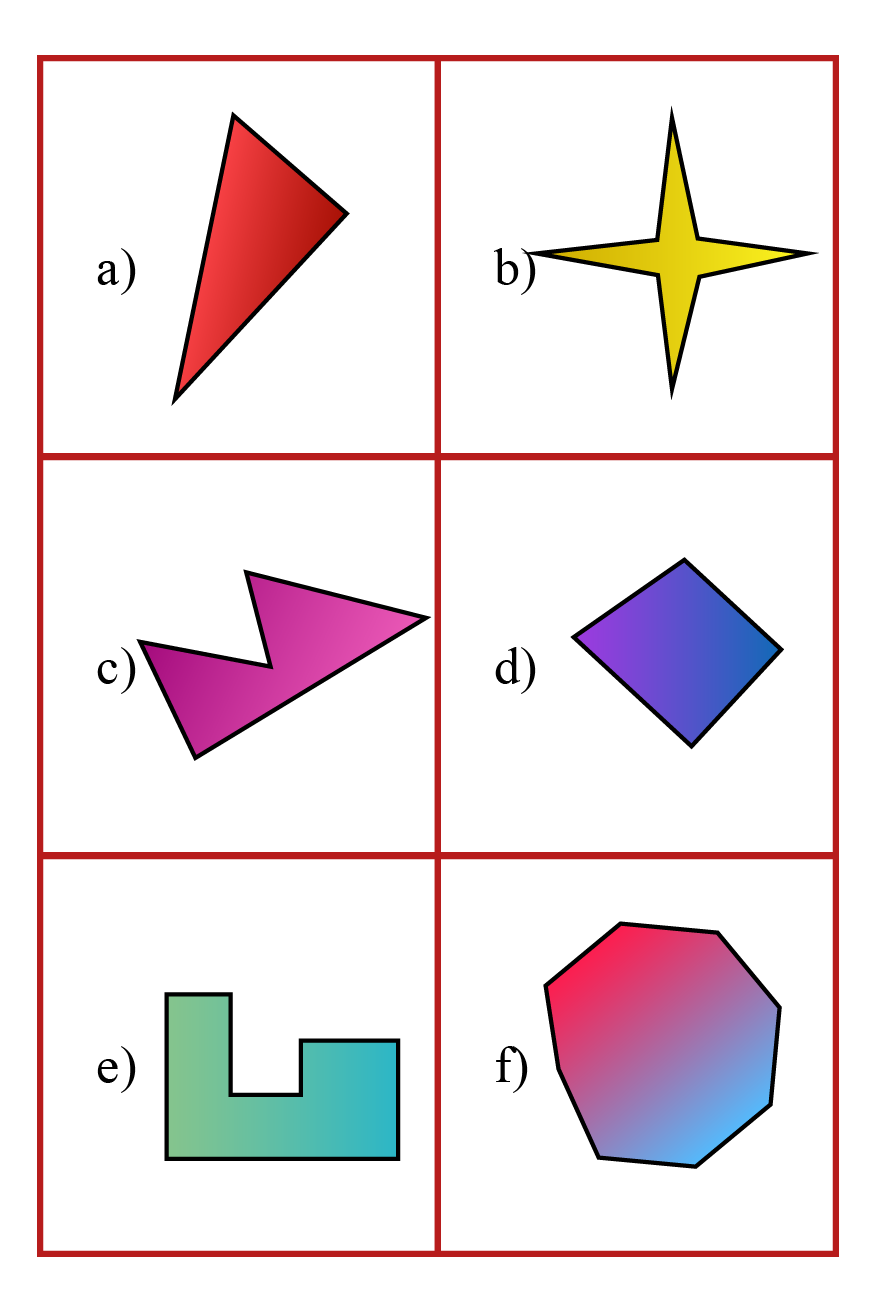Solution:

Among the given shapes, (a), (d), and (f) have all their vertices pointing outwards.

Also in (a), (d), and (f), all the interior angles measure less than $$180^\circ$$.

Therefore, these are the only convex shapes among the given shapes.

 Convex shapes are (a), (d), and (f).
 Example 3

Find the measure of each exterior angle of a regular nonagon.

Solution:

The number of sides of a nonagon is $$9$$

We know that the sum of all exterior angles of any convex polygon is $$360^\circ$$.

Since the given nonagon is regular, all the exterior angles measure the same.

Thus, each exterior angle of a regular nonagon is:

$\dfrac{360^\circ}{9}=40^\circ$

 Required angle = $$40^\circ$$

More Important Topics
Numbers
Algebra
Geometry
Measurement
Money
Data
Trigonometry
Calculus
More Important Topics
Numbers
Algebra
Geometry
Measurement
Money
Data
Trigonometry
Calculus